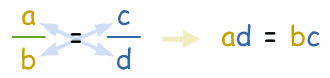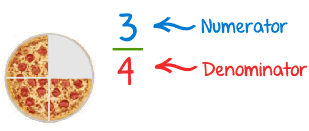# how to do cross multiplication

Cross multiplication is a method to compare two unlike fractions.

Suppose we want to compare two fractions.

At first, we will find the product of the numerator of first and denominator of the second (P1) and then find the product of the denominator of first and numerator of the second (P2).

Then on comparing both the products we can compare the given fractions.

If P1 > P2 , Then first fraction > second fraction

If P1 < P2, then first fraction < second fraction

If  P1 = P2, then first fraction = second fraction

suppose we have to compare 3/5 and 2/9.

Then P1 = 3 × 9 = 27

P2 = 5 × 2 = 10

Since P1 > P2 , so 3/5>2/9.

• 0
Cross Multiply

To cross multiply is to go from this:8=2123To this:8 × 3 = 12 × 2How Does it Work?

Step 1: When you multiply the topandbottom of a fraction by the same amount, it doesn't change its value.

Example (first fraction above):8=8× 31212× 3

In that example I multiplied the top and bottom of the first fractionby the bottom number of the second fraction.

Step 2: We could also multiply the top and bottom of thesecondfraction by the bottom number of thefirstfraction.

Example (second fraction above):2=2× 1233× 12

Step 3: And we would then have:

8× 3=2× 1212× 33× 12

And Magic! The bottom ofbothfractions is now12 × 3... !

Step 4: We can get rid of the12 × 3(because we are dividing both sides by the same amount) and the equation is still true:

8 × 3 = 12 × 2

Job Done!

In practice, though, it is easier to skip the steps and go straight to the "cross-multiplied" form.

Using Variables

So far I have used numbers, but we can state it more generally using variables:

To cross multiply is to go from this:a=cbdTo this:ad = bc

How to remember: "cross" multiply:Example

Cross multiplication can help speed up a solution. Like in this example:

Find "x":

x=28xLet's cross multiply:x2= 8 × 2 = 16And solvex = 4 or -4Terminology

I have been saying "top" and "bottom" of the fractions ... but the correct words arenumeratoranddenominator, OK?

(I just wanted to keep it simple.)

• 0
What are you looking for?